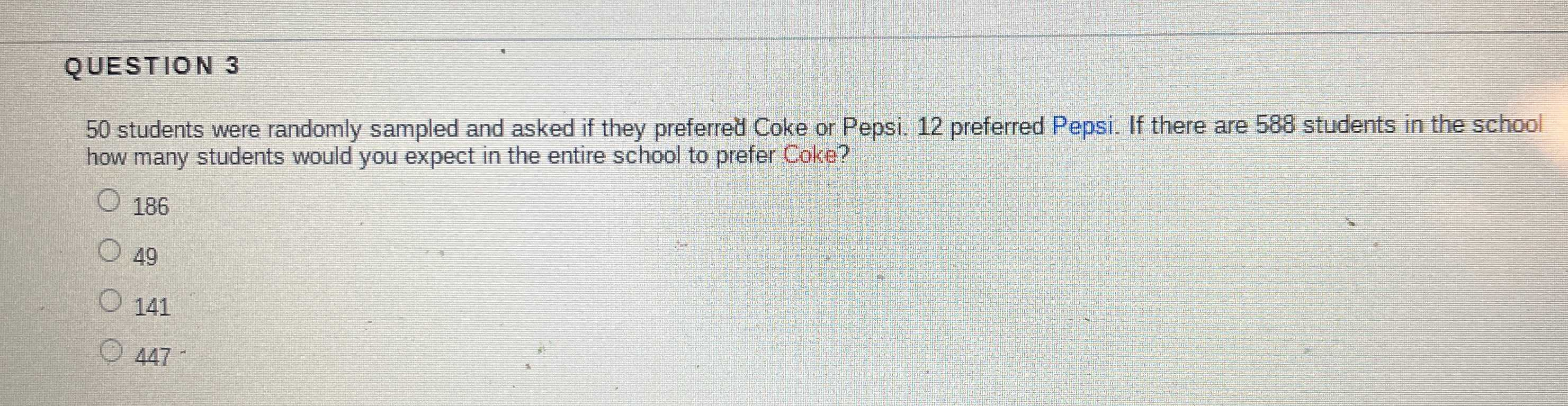### ¿Todavía tienes preguntas de matemáticas?

Pregunte a nuestros tutores expertos
Algebra
Pregunta$$50$$ students were randomly sampled and asked if they preferred Coke or Pepsi. $$12$$ preferred Pepsi. If there are $$588$$ students in the school how many students would you expect in the entire school to prefer Coke?

$$186$$

$$49$$

$$141$$

$$447$$